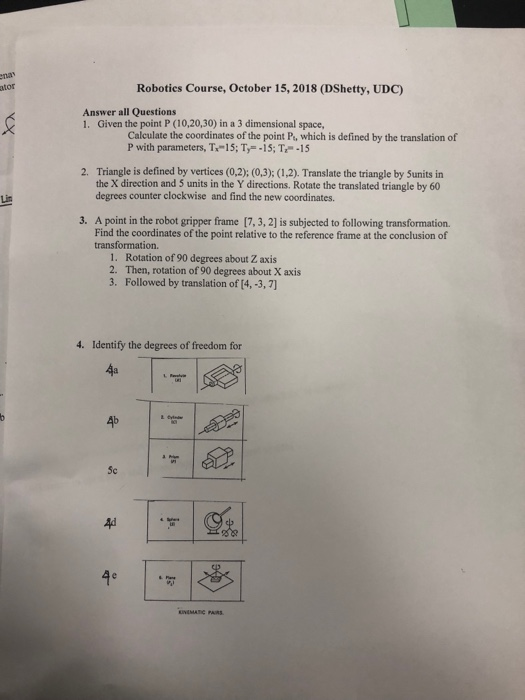# Question ator Roboties Course, October 15, 2018 (DShetty, UDC) Answer all Questions 1. Given the point P (10,20,30) in a 3 dimensional space, Calculate the coordinates of the point Pt, which is defined by the translation of P with parameters, T-15; T-15; T-15 Triangle is defined by vertices (0,2); (0,3): (1,2). Translate the triangle by Sunits in the X direction and ator Roboties Course, October 15, 2018 (DShetty, UDC) Answer all Questions 1. Given the point P (10,20,30) in a 3 dimensional space, Calculate the coordinates of the point Pt, which is defined by the translation of P with parameters, T-15; T-15; T-15 Triangle is defined by vertices (0,2); (0,3): (1,2). Translate the triangle by Sunits in the X direction and 5 units in the Y directions. Rotate the translated triangle by 60 degrees counter clockwise and find the new coordinates. 2. 3. A point in the robot gripper frame [7,3, 2] is subjected to following transformation Find the coordinates of the point relative to the reference frame at the conclusion of transformation. 1. 2· 3. Rotation of 90 degrees about Z axis Then, rotation of 90 degrees about X axis Followed by translation of 14,-3,7] 4. Identify the degrees of freedom for 4a 5c 의. 4e NEMATIC PAIRSCZ2LUN The Asker · Mechanical EngineeringTranscribed Image Text: ator Roboties Course, October 15, 2018 (DShetty, UDC) Answer all Questions 1. Given the point P (10,20,30) in a 3 dimensional space, Calculate the coordinates of the point Pt, which is defined by the translation of P with parameters, T-15; T-15; T-15 Triangle is defined by vertices (0,2); (0,3): (1,2). Translate the triangle by Sunits in the X direction and 5 units in the Y directions. Rotate the translated triangle by 60 degrees counter clockwise and find the new coordinates. 2. 3. A point in the robot gripper frame [7,3, 2] is subjected to following transformation Find the coordinates of the point relative to the reference frame at the conclusion of transformation. 1. 2· 3. Rotation of 90 degrees about Z axis Then, rotation of 90 degrees about X axis Followed by translation of 14,-3,7] 4. Identify the degrees of freedom for 4a 5c 의. 4e NEMATIC PAIRS
More
Transcribed Image Text: ator Roboties Course, October 15, 2018 (DShetty, UDC) Answer all Questions 1. Given the point P (10,20,30) in a 3 dimensional space, Calculate the coordinates of the point Pt, which is defined by the translation of P with parameters, T-15; T-15; T-15 Triangle is defined by vertices (0,2); (0,3): (1,2). Translate the triangle by Sunits in the X direction and 5 units in the Y directions. Rotate the translated triangle by 60 degrees counter clockwise and find the new coordinates. 2. 3. A point in the robot gripper frame [7,3, 2] is subjected to following transformation Find the coordinates of the point relative to the reference frame at the conclusion of transformation. 1. 2· 3. Rotation of 90 degrees about Z axis Then, rotation of 90 degrees about X axis Followed by translation of 14,-3,7] 4. Identify the degrees of freedom for 4a 5c 의. 4e NEMATIC PAIRS